# Prove (or disprove) that the $n^\text{th}$ derivative of $x^x$ at $x=1$ is a multiple of $n$.

First email

Subject: [Brilliant] New feedback program for problem creators

Hey <Author>,

We greatly appreciated your recent problems for their originality and creativity. We think you have great potential to further enhance your write-ups and have created a program for authors like you. In this, you will get our personalized feedback on your future problems about their completeness and clarity with suggestions like best theme or options for the problem. This will help your problems to stand out from the crowd and guide you to become a great author. To benefit fully from the experience, we ask you to devote about 3 hours of your time each week for a month.

If you’re interested in participating in September, reply YES to this email. To get started, you can read this Wiki page that explains the attributes of a great problem - Tempting to answer, Easy to get started, Imagery and Familiarity with setup - and use them to help your problems get popular.

Thanks, Brilliant Staff

Second email: For a positive response

Hey <Author>,

Thanks for showing interest in joining us to improve your write-ups and problem writing skills. You are a step closer towards becoming a great problem writer.

To get started, submit your future problem write-ups through this form. We will then reply to you in our chat room (details below) with feedback on how to improve the quality, correctness, and phrasing of your problem. This will allow you to update your write-up and post a great problem.

Chat room: Slack Brilliant Lounge is our chat room for communicating with other Brilliant members. Within the next day, you will receive an email from Slack with the subject of “Brilliant Lounge Bot has invited you to join Brilliant Lounge on Slack”. It will provide details on how to activate your account and join the chatroom.

Thanks, Brilliant staff.

Email for “if they say no” response

Thanks for responding. If you wish to join the problem in a later month, simply let us know.

We appreciate the problems that you’ve been creating and look forward to more of them.

Thanks, Brilliant staff.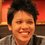Note by Pi Han Goh
6 years, 2 months ago

This discussion board is a place to discuss our Daily Challenges and the math and science related to those challenges. Explanations are more than just a solution — they should explain the steps and thinking strategies that you used to obtain the solution. Comments should further the discussion of math and science.

When posting on Brilliant:

• Use the emojis to react to an explanation, whether you're congratulating a job well done , or just really confused .
• Ask specific questions about the challenge or the steps in somebody's explanation. Well-posed questions can add a lot to the discussion, but posting "I don't understand!" doesn't help anyone.
• Try to contribute something new to the discussion, whether it is an extension, generalization or other idea related to the challenge.
• Stay on topic — we're all here to learn more about math and science, not to hear about your favorite get-rich-quick scheme or current world events.

MarkdownAppears as
*italics* or _italics_ italics
**bold** or __bold__ bold
- bulleted- list
• bulleted
• list
1. numbered2. list
1. numbered
2. list
Note: you must add a full line of space before and after lists for them to show up correctly
paragraph 1paragraph 2

paragraph 1

paragraph 2

[example link](https://brilliant.org)example link
> This is a quote
This is a quote
    # I indented these lines
# 4 spaces, and now they show
# up as a code block.

print "hello world"
# I indented these lines
# 4 spaces, and now they show
# up as a code block.

print "hello world"
MathAppears as
Remember to wrap math in $$ ... $$ or $ ... $ to ensure proper formatting.
2 \times 3 $2 \times 3$
2^{34} $2^{34}$
a_{i-1} $a_{i-1}$
\frac{2}{3} $\frac{2}{3}$
\sqrt{2} $\sqrt{2}$
\sum_{i=1}^3 $\sum_{i=1}^3$
\sin \theta $\sin \theta$
\boxed{123} $\boxed{123}$

## Comments

Sort by:

Top Newest

$\large \int _{ 0 }^{ \infty }{{ x }^{ 4 } \left( \int _{ 0 }^{ x }{ \frac { \sin t }{ t } \, dt } \right) \, dx }$

If the value of the integral above is equal to $- \dfrac AB$, where $A$ and $B$ are coprime positive integers, find $A+B$.

This integral does not converge. We are asked to integrate $\int_0^\infty x^4 \left(\int_0^x \frac{\sin t}{t}\,dt\right) \,dx$ The integrand $f(x) \; = \; x^4 \left(\int_0^x \frac{\sin t}{t}\,dt\right)$ exists for all $x$, but $f(x) \,\sim\, \tfrac12\pi x^4$ as $x \to \infty$, and hence $f(x)$ cannot be integrable on $[0,\infty)$.

Sir can you prove that the solution obtained by ramanujan's master theorem is wrong?

Whether $x^4$ is inside or outside the inner integral is irrelevant; it does not change the situation.

Ramanujan's Master Theorem needs to be used carefully. It does not work for just any function. Ramanujan's initial presentation of this Theorem was based on a series of formal arguments, which G.H.Hardy pointed out, when he proved the Theorem initially, were often invalid.

Basically, Ramanujan's Master Theorem is a formal identity, which is only true for certain types of functions. True, many functions are included in the requirements of the Theorem, but these requirements are such as to make the integrals converge! By definition, therefore, your function cannot satisfy the Theorem. For example, here is one formal statement of RMT, based on properties of the Mellin transform:

Let $\phi$ be a function which is analytic on $\{ z \in \mathbb{C}\,:\, \mathrm{Re} z \ge -\delta\}$ for some $0 < \delta < 1$. Suppose that, for some $A < \pi$ and $C,P > 0$, the function $\phi$ satisfies the growth condition $\big| \phi(v + iw) \big| \; \le \; Ce^{Pv + A|w|} \qquad \qquad v \ge -\delta\,,\,w \in \mathbb{R} \;.,$ Then $\int_0^\infty x^{s-1} \left(\sum_{n=0}^\infty (-1)^n\phi(n)x^n\right)\,dx \; = \; \tfrac{\pi}{\sin s\pi} \phi(-s) \;, \qquad \qquad 0 < \mathrm{Re}\,s < \delta \;.$

I suspect that your function does not satisfy this condition.

Ultimately, the region of validity of RMT (the values of $s$ for which it is true are basically the values of $s$ for which the ingegral on the LHS converges. If the integral $\int_0^\infty x^{s-1} \left(\sum_{n=0}^\infty (-1)^n\phi(n)x^n\right)\,dx$ converges, then the RMT can probably be used to evaluate it. It cannot, however, be used to give values to nonconvergent integrals.

You can integrate the Sine integral, but more care is needed. It can be shown, for example, that $\int_0^\infty x^{s-1}\left(\int_0^x \frac{\sin t}{t}\,dt - \tfrac12\pi\right)\,dx \; = \; -\frac{\Gamma(s)}{s} \sin(\tfrac12 \pi s) \;, \qquad \qquad 0 < \mathrm{Re}\,s < 1 \;.$ It is most likely that the RMT can be used to establish this one! However, the range of validity of $s$ does not include $s=5$, which is what I think you used to get your answer of $-\tfrac{4!}{5}$.

Formal manipulations need to be handled with care; Ramanujan, for example, manipulated series formally and came up with $1 + 2 + 3 + 4 + \cdots \; = \; -\tfrac{1}{12} \;.$ While it is true that $\zeta(-1) = -\tfrac{1}{12}$, and $1 + 2 + 3 + \cdots$ is what we obtain if we formally substitute $s=-1$ into the definition of $\zeta(s)$, that does not get around the fact that the series does not converge, to $-\tfrac{1}{12}$ or anything else. Of course, it has to be said that high-powered quantum physicists use this and similar formulae every day, but that is only as a short-hand for performing the analytic continuation analysis required to obtain $\zeta(-1)$ from the series formula for $\zeta(s)$ (which is only valid for $\mathrm{Re}\,s > 1$).Image

This is the original proof provided by Ramanujan. So you meant to say that there are some functions $\phi \left( x \right)$ the summations don't converge?

Even I was surprised that $1+2+3+4+...=\frac{1}{12}$. Is there any theoretical proof that it is wrong? Obviously practically anyone can prove that it is wrong.

Talking about this problem it would be best to delete it and post a new one.

Thanks for the explanation!

Can you provide a good source where I can properly learn how to use RMT?

I am quoting G.H.Hardy, who said that some of applications in the original paper were inapplicable. The scale of the graphic is pretty small, but Corollary 1 is the result we have been talking about, but the nature of the function $\phi$ is not specified. That is just was I was talking about; until the type of function is specified, this is just a formal statement. I believe that some of the cases that Ramanujan gave as examples of his idea were based on functions $\phi$ for which the result was not applicable.

Minus $\tfrac1{12}$, not plus $\tfrac{1}{12}$. The series diverges to infinity - what more proof is needed? The theory of complex functions is strange; the fact that the zeta function has a perfectly reasonable value at $-1$ which has, on the face of it, nothing whatsoever to do with the formulae defining the zeta function elsewhere is one of the weird consequences of the theory of analytic continuation.

I am afraid there is no real way to redeem your question.

- 5 years, 3 months ago

Log in to reply

This is something of a special case of Euler's work on the generating function of the partition function $P$.

Let us let $S(n)$ be the sum $S(n) \; =\; \sum_{{a_1,a_2,\ldots,a_n \ge0} \atop {a_1,a_2,\ldots,a_n \text{ distinct}}} 2^{-(a_1+a_2+\cdots+a_n)} \;= \; n! \sum_{0 \le a_1 < a_2 < \cdots < a_n} 2^{-(a_1+a_2+\cdots+a_n)} \;,$ Then $S(n) \; = \; n! \sum_{N \ge \frac12n(n-1)} \big|A(N,n)\big| 2^{-N} \;,$ where $A(N,n)$ is the set $A(N,n) \; = \; \left\{ \mathbf{a} \in \mathbb{N}\cup\{0\}^n \; \Big| \; a_1 < a_2 < \cdots < a_n \;, \; \sum_{j=1}^n a_j \,=\, N \right\} \;, \; N \ge \tfrac12n(n-1) \;.$ If we also consider the set $B(L,n) \; = \; \left\{ \mathbf{b} \in \mathbb{N}\cup\{0\}^n \; \Big| \; \sum_{j=1}^n (n+1-j)b_j \,=\, L\right\}\;, \qquad L \ge 0 \;,$ then the mapping $X \,:\, B\big(N-\tfrac12n(n-1),n\big) \,\to\, A(N,n)$ given by the formula $(X\mathbf{b})_j \; = \; j-1 + \sum_{r=1}^j b_r \qquad 1 \le j \le n \;, \qquad \qquad \mathbf{b} \in B\big(N-\tfrac12n(n-1),n\big)$ is a bijection for any $N \ge \tfrac12n(n-1)$, and hence $\big|A(N,n)\big| \,=\, \big|B(\big(N-\tfrac12n(n-1),n\big)\big|$.

But $\big|B(L,n)\big|$ is the coefficient of $t^L$ in the series expansion of the product $\prod_{r=1}^n \big(1 - t^r\big)^{-1} \;,$ and hence $\big|A(N,n)\big|$ is the coefficient of $t^N$ in the series expansion of the product $Q_n(t) \; = \; t^{\frac12n(n-1)}\prod_{r=1}^n \big(1 - t^r\big)^{-1} \; = \; \prod_{r=1}^n \left(\frac{t^{r-1}}{1-t^r}\right) \;.$ Thus it follows that $S(n) \; = \; n! Q_n(\tfrac12) \; =\; n! \prod_{r=1}^n \frac{2}{2^r-1} \; = \; n!2^n \left(\prod_{r=1}^n (2^r-1)\right)^{-1} \;.$ In this case, we have $S(3) \; =\; \frac{3! \times 2^3}{1\times3\times7} \; =\; \frac{16}{7} \;,$ making the answer $16+7 = \boxed{23}$.

I have so many questions and I don't know where to begin, so let me just ask whatever that appears the most confusing:

Question 01: How did you convert this first $S(n)$ to the second $S(n)$?

$S(n) \; =\; \sum_{{a_1,a_2,\ldots,a_n \ge0} \atop {a_1,a_2,\ldots,a_n \text{ distinct}}} 2^{-(a_1+a_2+\cdots+a_n)} \;= \; n! \sum_{0 \le a_1 < a_2 < \cdots < a_n} 2^{-(a_1+a_2+\cdots+a_n)} \;,$

to

$S(n) \; = \; n! \sum_{N \ge \frac12n(n-1)} \big|A(N,n)\big| 2^{-N} \;,$

(Yes, I've read your definition of $A(N,n) = \ldots$ already and I'm still clueless)

Question 02: what does $\mathbf{a} \in \mathbb{N}\cup\{0\}^n$ means? Does it mean a vector called $a$, with all non-negative integer elements right?

Question 03: Can you explain to me what this means? Mapping? Do you mean a one-to-one relation?

then the mapping $X \,:\, B\big(N-\tfrac12n(n-1),n\big) \,\to\, A(N,n)$ given by the formula

Question 04: What does $\displaystyle(Xb)_j \; = \; j-1 + \sum_{r=1}^j b_r$ means? What $(Xb)_j$ means exactly? I didn't see this notation before in your solution.

1. Every term $2^{-a_1-a_2-\cdots-a_n}$ with $a_1+a_2+\cdots +a_n = N$ contributes $2^{-N}$ to the sum. The number $|A(N,n)|$ just counts how many different times $2^{-N}$ occurs in the sum. Basically, I am collecting terms.

2. Yes. $\mathbb{N}\cup\{0\}^n$ is the set of $n$-tuples of nonnegative integers.

3. A mapping is another word for a function. Since I am saying that $X$ is a bijection, you can read this whole bit as saying that $X$ sets up a 1-1 correspondence between the two sets, if that is a more familiar expression to you.

4. Remember, $X$ is a function between sets of $n$-tuples. If we start with the $n$-tuple $\mathbb{b}$ in $B(N-\tfrac12n(n-1),n)$, then $X\mathbf{b}$ is the $n$-tuple (call it $\mathbf{a}$) whose coefficients are $a_j \; = \; j - 1 + \sum_{r=1}^j b_r \;, \qquad 1 \le j \le n \;.$ I am using the standard notational abbreviation $\mathbf{a}$ to represent the $n$-tuple $(a_1,a_2,\ldots,a_n)$, and similarly for $\mathbf{b}$. You need to check that $X\mathbf{b}$ actually belongs to $A(N,n)$ (that is the point of $X$) and that $X$ has an inverse, so that every element of $A(N,n)$ is equal to $X\mathbf{b}$ for a unique element $\mathbf{b}$ in $B(N-\tfrac12n(n-1),n)$. You retrieve $\mathbf{b}$ from $\mathbf{a} = X\mathbf{b}$ by the formula $b_j \; = \; \left\{ \begin{array}{lll} a_1 & \qquad \qquad j = 1 \\ a_j - a_{j-1} - 1 & \qquad \qquad 2 \le j \le n. \end{array} \right.$

Thank you for your reply. Even if I accept that the function $A(N,n)$ is carefully constructed, I still couldn't for the life of me figure out how you managed to pull the function of $B(L,n)$ out of nowhere. That's some outstanding detective work!

Will read your solution again once my head is not about to explode.

There are standard combinatoric tricks for converting sets of strictly increasing $n$-tuples into sets of just increasing $n$-tuples, or even (as I did in this case) into sets of $n$-tuples of nonnegative numbers. Generally speaking, it is easier to identify what a set of $n$-tuples is doing if there are as few restrictions on the shape of its elements as possible; going from $a_1 < a_2 < \cdots < a_n$ to $b_1,b_2, \ldots b_n \ge 0$ was a big simplification, and it seemed to me worth the while (to be more accurate, I have read about how to derive the generating function for the partition function $P$, and this calculation is similar), to investigate what would happen if we made this conversion. As you can see, it worked!

Where did I get $B(L,n)$ from? It was forced on me. The formula $b_j \; = \; \left\{ \begin{array}{lll} a_1 & \qquad \qquad & j = 1 \\ a_j - a_{j-1} - 1 && 2 \le j \le n \;. \end{array} \right.$ is one of those standard tricks for converting an $n$-tuple $\mathbf{a}$ with $a_1 < a_2 < \cdots < a_n$ into an $n$-tuple $\mathbf{b}$ with $b_1,b_2,\ldots,b_n \ge 0$. Check out what the requirement that the components of $\mathbf{a}$ add to $N$ has on the components of $\mathbf{b}$, and out pops the sum requirement of $B(L,n)$, for $L = N - \tfrac12n(n-1)$.

- 5 years, 3 months ago

Log in to reply

This integral fails to converge. If we attempt to evaluate the infinite integral using polar coordinates, we obtain $\begin{array}{rcl} \displaystyle \int\int_{{x,y \ge0 \atop x^2 + y^2 \le R^2}} \sin(x^2+y^2)\,dx\,dy & = & \displaystyle \int_0^{\frac12\pi} \int_0^R \sin r^2\, r\,dr\,d\theta \\ & = & \displaystyle \tfrac12\pi\int_0^R r \sin r^2\,dr \\ & = & \displaystyle \tfrac12\pi \Big[-\tfrac12 \cos r^2 \Big]_0^R \\ & = & \displaystyle \tfrac14\pi\big(1 - \cos R^2\big) \end{array}$ which has no limit as $R \to \infty$.

That we can calculate the infinite integral $\int_0^\infty \sin(x^2+y^2)\,dx \; = \; \int_0^\infty \big(\sin x^2 \cos y^2 + \cos x^2 \sin y^2\big)\,dx \; = \; \sqrt{\frac{\pi}{8}}\big(\sin y^2 + \cos y^2\big)$ so that the iterated integral $\int_0^\infty \left( \int_0^\infty \sin(x^2 + y^2)\,dx\right)\,dy \; = \; \tfrac14\pi$ exists (which may be the author's intention) is not relevant.

Wait.... I did your second method to get $\dfrac \pi4$. What's wrong with it?

Or why is there conflicting results when we do polar coordinates? Did I commit some sort of fallacy?

I ask the question back at you. If you are correct, and the integral exists, what is wrong with my calculation using polar coordinates?

The existence of the iterated integral does not imply the existence of the double integral. Particularly so in a case like this, where both stages of the iteration involve infinite Riemann integrals which do not converge in a Lebesgue sense.

This is one of the strengths of Lebesgue integration over Riemann integration; if the double integral exists, then the iterated integral must also exist, and equal the double integral. This works for infinite integrals.

With Riemann integration, we only have a similar result for integrals on bounded areas.

The downside of the strength of Lebesgue integration, theory-wise, is that not so many functions are Lebesgue-integrable. The function $\sin x^2$ is not Lebesgue-integrable on $\mathbb{R}$, for example. However, Lebesgue integration theory can handle the Fresnel integrals just as well as Riemann integration, but at the expense of greater notational care. Instead of just writing $\int_0^\infty \sin x^2\,dx \; = \; \sqrt{\tfrac{\pi}{8}}$ as Riemann integration would do, we would have to write $\lim_{R\to\infty}\int_0^R \sin x^2\,dx \; = \; \sqrt{\tfrac{\pi}{8}}\;.$ This is, of course, exactly what Riemann integration means, but it is more careful. When Lebesgue integration uses the integral sign, we can be much more confident about various manipulations that we can perform with it - for example, we have the ability to convert an iterated integral to a double one.

It took me several hours to understand what you're saying, but I don't think I understood it completely. Maybe because I did not (formally) learn what Lebesgue integral is.

The existence of the iterated integral does not imply the existence of the double integral.

This is the first time I hear it. I learn some basic calculus (mostly James Stewart textbook) back in the days, but I don't think I ever come across this line before. It sounds so counterintuitive. If what you said is true (no, I do not doubt you, I'm just shocked), then why didn't my math education ever taught me this basic calculus fact? I'm just venting at this point because I'm disappointed that I didn't learn calculus correctly. As a professor (I assume you are one), do you have any thoughts about this flawed education system?

You completely lost me at this part: "Convert $\displaystyle \int_0^\infty \cdots$ to $\displaystyle \lim_{R\to\infty} \int_0^R \cdots$". Aren't they same thing?! (Emphasized that I'm puzzled and shouting because I was caught off guard) Are you saying that at least one of the following equations is/are incorrect (or not necessarily true)? $\text{Equation one: } \qquad \int_0^\infty \int_0^\infty \sin(x^2+y^2) \, dx \; dy = \lim_{R\to\infty} \int_0^R \int_0^R \sin(x^2+y^2) \, dx \; dy$

$\text{Equation two: } \qquad \int_0^\infty \int_0^\infty \sin(x^2+y^2) \, dx \; dy = \lim_{R_1 \to\infty} \lim_{R_2\to\infty} \int_0^{R_1} \int_0^{R_2} \sin(x^2+y^2) \, dx \; dy$

Or worse come to worst, could you either direct me to a useful textbook that explains all these? (I have Rudin but I felt that it's too complicated for a simpleton such as myself) Or (if it's possible) could you simplify what you said without getting too technical? Because I don't fully understand all the terms like "Lebesgue-integrable", "Lebesgue integration".

(Note: I don't find wikipedia helpful because they are not really engaging to newbies as such myself)

I appreciate your swift and detailed reply (every time). This site needs more people like you. Huge respect.

This is going to be huge.

Riemann integration in 1D is only really defined for bounded intervals. Infinite integrals are fudged by saying that a function can be integrated over an infinite interval if limit of the integrals over finite intervals exists as those intervals "tend to infinity" by any method possible. Thus we can say (in the Riemann sense) $\int_0^\infty f(x)\,dx \; = \; \lim_{R\to\infty} \int_0^R f(x)\,dx$ because the limit on the right basically encompasses all the ways that a finite interval can expand to include all positive numbers. However we cannot define $\int_{-\infty}^\infty f(x)\,dx \; = \; \lim_{R\to\infty} \int_{-R}^R f(x)\,dx \;,$ since there are more ways of letting a finite interval tend to infinity. We have to define $\int_{-\infty}^\infty f(x)\,dx \; = \; \lim_{X,Y \to \infty} \int_{-X}^Y f(x)\,dx$ with both $X$ and $Y$ tending to infinity at the same time. This is different to the first statement, since it allows for the possibility of $X$ and $Y$ tending to infinity at different rates. For example, we would not want to claim that $\int_{-\infty}^\infty x\,dx \; = \; 0$ even though the integrals from $-R$ to $R$ all exist and are $0$; the integrals from $-R$ to $2R$ diverge to $\infty$, for example, and the integrals from $-2R$ to $R$ diverge to $-\infty$.

If we move on to 2D integration, the first thing to note is that it is defined independently of 1D integration. The integral of a function of two variables over an interval is not defined as either of the iterated integrals. It is too long to write out the theory of Riemann integration in 1 or 2 dimensions here. It is a theorem that, for suitably well-behaved functions $f$, then the integral of $f$ over a rectangle is equal to the iterated integral, but this is a nontrivial result.

Just as 1D Riemann integration is defined for finite intervals, and extended to infinite intervals by a bit of a fudge, infinite 2D integrals have to be obtained as limits in the same way. Without going into all the details, for the integral $\int_0^\infty \int_0^\infty f(x,y)\,dx\,dy$ to exist, all of the limits $\lim_{R \to \infty} \int_0^R \int_0^R f(x,y)\,dx\,dy \quad \lim_{R_1,R_2\to\infty}\int_0^{R_1}\int_0^{R_2} f(x,y)\,dx\,dy \quad \lim_{R \to \infty} \iint_{{x,y \ge 0} \atop {x^2 + y^2 \le R^2}} f(x,y)\,dx\,dy$ would have to exist and be equal, as well as any other limit of integrals over finite regions which eventually cover the whole first quadrant.

To be honest, people get lazy with Riemann integration, and forget about the technicalities of the limiting process. The limits are omitted from the integrals, and this can result in people assuming that certain deductions can be made which are not valid. A careful use of Riemann integration is always aware of the problems of infinite integrals.

That is where Lebesgue integration comes in. This is a different formulation of integration theory. It is very similar to Riemann integration, but is structured in a subtly different manner. This has a number of very positive consequences:

• the Lebesgue integral can be defined on infinite intervals (or infinite regions in 2D) just as easily as on finite intervals
• if a function $f(x)$ is Lebesgue integrable, so is $|f(x)|$, with the expected relationship between the sizes of their integrals (this is not true of the Riemann integral),
• a swathe of powerful theorems can be proved about these integrals, which do not hold for Riemann integrals.
• in particular, if a function is integrable over a region, then the two iterated integrals both exist, and are equal to the integral of the function.

The downside is that fewer functions are Lebesgue-integrable. However, when a Lebesgue integration theorist talks about the integral $\int_0^\infty f(x)\,dx$ she is not talking about a cute limit of finite integrals, but about something that is naturally defined on the whole infinite integral, and which therefore can benefit from all the advantanges I listed above.

If we look at an "identity" like $\int_0^\infty f(x)\,dx \; = \; \lim_{R \to \infty} \int_0^R f(x)\,dx \,$ then this can be read in diferrent ways:

• from an Riemann integration perspective, this would be the definition of the infinite integral.
• if the function $f$ was Lebesgue-integrable on $(0,\infty)$, this identity would be a true statement, and a consequence of one of those nice theorems of Lebesgue integration.
• if the function $f$ was not Lebesgue-integrable on $(0,\infty)$, then the LHS would make no sense. The RHS might still make sense (try $f(x) = \frac{\sin x}{x}$), but that would still not make the function Lebesgue-integrable.

I know this is jumping around a lot, but here is a pretty standard example of a function for which evaluating the iterated integrals does not help. The integrals $\int_0^1 \left(\int_0^1 \frac{x^2-y^2}{(x^2+y^2)^2}\,dx\right)\,dy \qquad \int_0^1 \left(\int_0^1 \frac{x^2-y^2}{(x^2+y^2)^2}\,dy\right)\,dx$ evaluate to $\pm\tfrac14\pi$; I leave it to you to determine which is which. Thus the two iterated integrals, although they both exist, tell us nothing about the integrability of this function over the square; this function is not even Riemann-integrable over the square.

- 5 years, 3 months ago

Log in to reply

The problem with this issue is that we find it difficult to get out heads around limits of series. Since these can be pretty weird, that is forgiveable!.

Let us assume that the number $a = 0.\dot{9}$ exists. Since $0.999\cdots9$ (for any finite number of $9$s) is less than $1$, we can reasonably deduce that $a \le 1$.

Suppose now that $0 < b < 1$. If we consider the decimal expansion of $b$, we will eventually find a number after the decimal point which is less than $9$. In other words, we will be able to write $b < 0.999\cdots9$, where the number on the right of this inequality has a finite number of $9$s after its decimal point. Thus $b < a$.

In other words the number $a$ is less than or equal to $1$, but is greater than any number less than $1$. This means that $a$ must be equal to $1$. In other words, if $0.\dot{9}$ exists, it must be equal to $1$.

The only problem left is that of accepting that the number $a$ exists in the first place. This is a nontrivial issue, and many mathematicians, starting with the ancient Greeks, have had problems with infinite limits. Consider such "paradoxes" as Zeno's paradox or the Achilles and the tortoise paradox.

If we are prepared to accept a mathematical system within which limits exist, then $0.\dot{9}$ will both exist and be equal to $1$, and $0.\dot{3}$ will exist and be equal to $\tfrac13$. The existence of such limits is based upon what is called the Completeness Axiom of the Reals; while mathematicians might try to see what can be deduced in the absence of this Axiom, I would think that few disbelieve it.

It is interesting that students find the existence of $0.\dot{9} = 1$ harder to accept than they do the existence of $0.\dot{3} = \tfrac13$. Accepting that either of these numbers exists involves coping with the idea of an infinite number of entries in a decimal expansion; there is something about the fact that the identity $0.\dot{9} = 1$ increases the digit before the decimal point that gets people agitated, while students at a very early age have few problems with $0.\dot{3} = \tfrac13$. From a teaching point of view, therefore, observing that $3 \times 0.\dot{3} \,=\, 0.\dot{9}$, and therefore that $0.\dot{9} = 3\times\tfrac13 = 1$ is an argument which can content most students, at least until they can get to grips with the proper definition of the limit of a series.

(Printer is on) hahaahahah! Just kidding!

You wrote what Otto Brestcher wrote up here in the solution discussion but in a much clearer manner!

Good read! I didn't think of the analogy between Tortoise paradox and $0.\overline9=1$.

Completeness Axiom of the Reals? Woah! First time hearing of about this! Good read! Your comments are always so valuable! Brilliant should always send me a notification wherever you post anything in Brilliant!!

Can you explain why this happens? Or why mathematicians disagree about this (Is it because most people can't grasp new concepts like the controversy over Cantor's theory)? Does this issue still occur? And why?

while mathematicians might try to see what can be deduced in the absence of this Axiom, I would think that few disbelieve it.

It is not so much a case of mathematicians disagreeing about it; the Completeness Axiom (i.e. the existence of least upper bounds) is what defines the reals. Mathematicians can, however, be interested in determining how much can be deduced without assuming this Axiom. An increasing bounded sequence converges to its least upper bound; if we drop the Completeness Axiom, then we cannot have limits of any sequences except those which are eventually constant (and for which convergence is obvious). No limits means no calculus, and the whole field of Analysis goes down the tubes. What you are left with is mathematics that can be done within the rationals - Diophantine equations and the like. These are, of course, exceptionally rich fields of study.

It is interesting to note how Analytic Number Theory has found great usefulness of the real number system, and the machinery of analysis of real number, in the service of solving equations and problems about rational numbers.

In a similar manner, logicians are interested in what can be deduced if you remove Proof by Contradiction from your armoury (this is an example of what is called Constructivist Logic). This is important, since computers cannot prove by contradiction, and so results that can be proved without using Proof by Contradiction are essentially those that can be machine-proved

I don't know much about this subject matter (of Completeness Axiom) until recently, so forgive me if I sound ignorant, but is it in any way similar to accepting/rejecting the Axiom of Choice? Because I'm more familiar with that area as compared to Completeness Axiom.

Mathematicians can, however, be interested in determining how much can be deduced without assuming this Axiom.

Can you explain to me why these mathematicians prefer to restrict themselves by not assuming this axiom? Because I don't see the benefit of it at all. Is it because they want to find a more elegant solution to the necessary questions? Or because they think that this Axiom is not rigorous (highly doubt it)? Or something else entirely?

From your entire first paragraph, it sounds to me that what you're saying is that some mathematicians prefer not to use Completeness Axiom simply because of they are merely asserting one's preference, no?

Wait, isn't proof by contradiction super duper important? Why are logicians interested in handicapping themselves by restricting themselves by not using that commonplace method? What's the point of all these? Is there a benefit to this restriction?

The Axiom of Completeness of the reals has nothing to do with the Axiom of Choice, except that they are both Axioms! The Completeness Axiom says that any bounded nonempty set of reals has a least upper bound, something which is not true about rationals. The Axiom of Choice states that it is possible (essentially) to make uncountably many selections at the same time - if I have a collection of sets $S_x$, one for each real number $x$, the AofC says I can create a function $f$ with domain $\mathbb{R}$ such that $f(x) \in S_x$ for all $x$. This Axiom permits transfinite induction (induction on uncountable sets). Much high level mathematics would be impossible without this Axiom. However, it is still an Axiom, in that we have no proof that it is true. Mathematics with and mathematics without the AofC are both perfectly consistent; maybe one day someone will discover a subtle difference between what is possible in both systems which will determine which is true!

Mathematics is the business of investigating what is possible. It can be interesting to investigate what can be deduced if only some properties, or methods of argument, are assumed. That is why mathematicians study groups, for example. There are many cases where a number system is more complex than being just a group, but knowing its group-theoretic properties is nonetheless interesting. Alternatively, Algebraic Number Theorists can be quite happy studying the integers, and do not always need to think about the reals at all ( on the other hand, Analytic Number Theorists have found great value in real number theory in the study of integers).

As I said previously, if you are a computer theorist, you are not that fond of Proof by Contradiction, since your pet machines cannot use it. You are therefore extremely interested in what can be proved without it. Mathematical Logic, without Proof by Contradiction, is perfectly consistent and sensible; the only problem is that there are lots of elementary statements that are true, but cannot be proved, within it. Of course, Godel's Incompleteness Theorem proves that there are true, but unprovable, statements within any sufficiently complex system of logic. The difference is that we don't ever know in general which those statements are, whereas it is easy to find true statements which cannot be proved without Contradiction.

Thanks for your immediate reply!

I spent some time last week to read up all these axioms again and to be honest, I don't fully grasp most of them. Maybe due to the extreme lack of exercises in the (only) textbook that I bought. Or that there are so many complicated "consequences" of this Axiom (of Choice), like Zorn's Lemma, Hausdorff's Maximal Principle.

Do you have any good books on Set Theory? Because without sufficient exercises, I will (still) find it hard to grasp these concepts. Same goes for any books that teach The Completeness Axiom, because I don't think wikipedia paints a good picture for newbies to understand it, nor can I find any good YouTube videos for it, and most of the other explanations that I can find appears very handwavy.

The classic text is P.R. Halmos' "Naïve Set Theory", but it is pretty tough-going.

Oh I bookmarked this and I forgot to reply.

Are you referring to this one? Okay! ordered!

Pretty tough-going? Even for you? Well, I guess I can only master this topic in the next decade.

Thanks for the dialog!

That's the one. It should get you started.

Thank you! You're really really helpful!!

- 5 years, 3 months ago

Log in to reply

Can you list some EASY functions to be listed in this page?

Please don't link to wikipedia again, it's hard to understand most of what they're saying.

I would start with a discussion of multiplicative functions, namely functions $f$ on $\mathbb{N}$ such that $f(mn) = f(m)f(n)$ whenever $m,n$ are coprime. Examples of these are legion! The identity function, the number of divisors function, the sum of divisors function, the Euler totient function, the Mobius function, the many functions that lurk in Brilliant Number Theory problems...

Then introduce the Dirichlet convolution $\star$, with the observation that $\star$ is commutative and associative, and the result that the convolution of two multiplicative functions is multiplicative. Since $\mu \star 1$ is multiplicative, it is easy to show that it is equal to $\delta$, where $\delta(n) \; = \; \left\{ \begin{array}{lll} 1 & \qquad & n = 1 \\ 0 & \qquad & n \ge 2 \;, \end{array} \right.$ is the multiplicative identity for the Dirichlet convolution. This is enough to show that if $f = g\star 1$, then $g = f \star \mu$, which is the Dirichlet inverse result.

At this point easy counting of HCFs shows that $\phi \star 1 = \mathrm{id}$, which gives us $\phi = \mu \star \mathrm{id}$, and we are away...

Don't introduce the Dirichlet convolution out of nowhere; put it in the context of multiplicative functions, with the convolution as a useful device for creating new multiplicative functions from old, and the reason for its existence/usefulness is more obvious.

Woah that's super detailed! Let me see if there's a way to improve that wiki page (as it is already written up).

Please tell me you've published books/autobiography before, I want to read everything a god (you) has to say!

- 5 years, 3 months ago

Log in to reply

Can you please post your solution here so I can print and frame it? (Pretty please)

My method was hard work (but I was using Mathematica to perform the algebra). I differentiated $\tfrac12B(\tfrac12(u+1),\tfrac12)$ four times, and put $u=0$, to obtain $a \; = \; \int_0^{\frac12\pi} \big[\ln(\cos x)\big]^4\,dx\; = \; \tfrac{19}{480}\pi^5 + \tfrac14\pi^3 (\ln2)^2 + \tfrac12 \pi (\ln2)^4 + 3\pi\ln2 \zeta(3) \;.$ I then differentiated the (manipulated) W&W formula twice with respect to $u$, putting $v=0$, to obtain $\int_0^{\frac12\pi} \big[ \ln(\cos x)\big]^2 \cos vx\, dx \;= \; \tfrac{1}{12v^3}\left[12 + 2\pi^2v^2 - 3\pi^2v^2\mathrm{cosec}^2(\tfrac12v\pi) + 3v^2\left(H_{-\frac12v} + H_{\frac12v} + 2\ln2\right)^2\right] \sin\tfrac12v\pi\;.$ I then differentiated this twice with respect to $v$ and put $v=0$ to get the second integral. $b \; = \; \int_0^{\frac12\pi} \big[\ln(\cos x)\big]^2 x^2\,dx \; = \; \tfrac{11}{1440}\pi^5 + \tfrac{1}{24}\pi^3 (\ln 2)^2 + \tfrac12\ln 2\zeta(3) \;,$ and $\begin{array}{rcl} a - 6b & = & \left(\tfrac{19}{480}\pi^5 + \tfrac14\pi^3 (\ln2)^2 + \tfrac12 \pi (\ln2)^4 + 3\pi\ln2 \zeta(3) \right) - \left( \tfrac{11}{240}\pi^5 + \tfrac{1}{4}\pi^3 (\ln 2)^2 + 3\pi\ln 2\zeta(3) \right) \\ & = & \tfrac12\pi(\ln 2)^4 - \tfrac{1}{160}\pi^5 \;. \end{array}$

Aditya's method of using the W&W formula is much better than mine, since the integral he obtains is much easier to differentiate multiple times. I made the mistake of working out the first integral quickly, and then focused on getting the second integral on its own, rather than obtaining the two together!

My concern with Aditya's method is not that it was not a good one (it was!), but that the key formula he wanted to use needed more proof than he gave it.

Hey, The problem with my method is that I have used the binomial theorem to prove the result for only positive integral values of $u$, right? Differentiation is not allowed in that case, and it was silly of me to overlook this. But, I am now editing the solution so that I use the binomial series, which converges for the given conditions, i.e. $(1+z)^{u} = \displaystyle\sum_{k=0}^{\infty}{{u}\choose{k}}z^k$, where $\displaystyle{{u}\choose{k}}$ is the generalized binomial coefficient. Since $|z| = 1$ and $u>-1$ the series converges for all $u$ taken, right? I hope this fixes my incomplete solution. Anyways, thanks for showing another amazing way how to do it!

It's still not going to be straightforward, since the series does not converge at $x=\tfrac12\pi$ when $u < 0$ (and you want to differentiate at $0$, so have to handle what is happening for negative $u$). Showing that you can integrate the series term-by-term is not automatic.

The series does converge uniformly in $x$ for all $u > 0$, and so we can calculate the integral for all $u > 0$ by your proposed method. We can then differentiate four times, and let $u$ tend to $0$, using continuity in $u$ of all the expressions involved, thanks to the DCT.

- 5 years, 3 months ago

Log in to reply

….

# Top of the page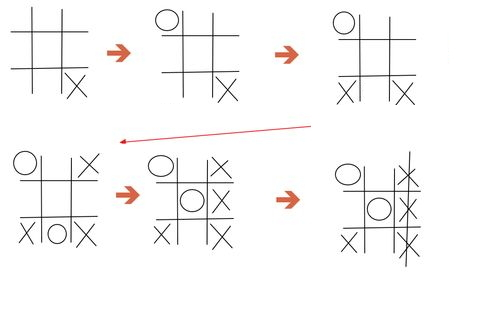(Need to convert to GiF)

Above shows a Tic Tac Toe game. Tic tac toe is a $2$-player game, where the players $X$ and $O$ take turns filling a $3 \times 3$ grid. Whoever places three respective marks in a vertical, horizontal, or diagonal manner will be the winner of the game. A draw is obtained whenever nobody wins. Like chess, a player needs good observation, tactics, and strategy to win a game of tic tac toe.

This wiki page is designed to help readers understand how to identify and avoid forks when playing a Tic Tac Toe.

In this page, we are making it consistent by displaying $\color{#D61F06}{X}$ as the first player and $\color{#3D99F6}{O}$ as the second player. The centre will be the centre of the grid (obviously). The corner will be the corner of the grid and the edge will be the squares adjacent to the corner and centre.

To avoid repetition of explanation, user should be able to identify puzzle that are similar simply because of rotations. For example, the pictures on the left are equivalent to the pictures on the right respectively.

This wiki page does not intend to list down all possible scenarios for forking, so you should take this page in the spirit that you should not memorize all the forking configurations, but ways of identifying and avoiding forking formations.After reviewing this page, you should be to know that if both played optimally, it will always forces a draw.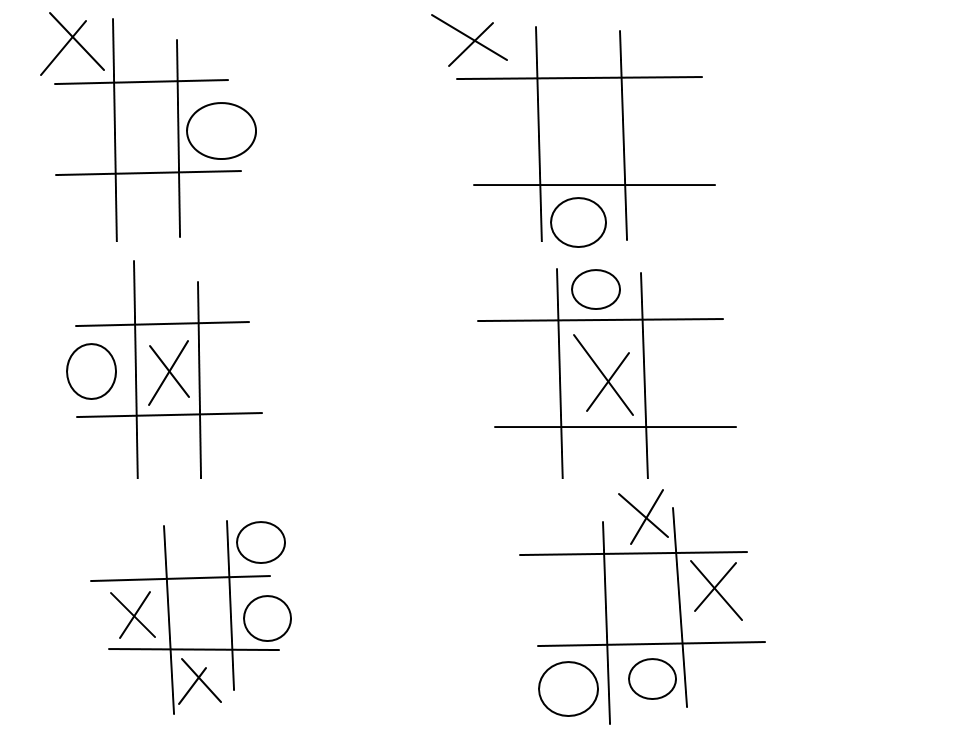Contents

# 1.Basic Rules and definition

...... 1.1 Rules
...... 1.2 Thinking one step ahead (see pic)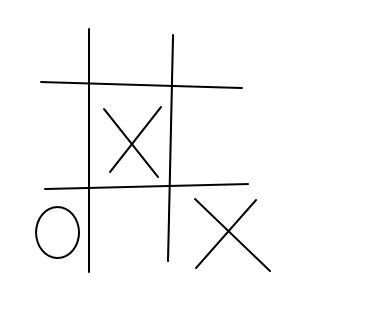Question: Where should player 2 make?
Answer: Obviously top left, else you lose the game.

(The colors were meant to color the entire cell)

…. 1.3 THREE sets of opening:::

Noting the symmetries, We first note that there will be 3 opening from player X... he/she can put in center/edge/corner.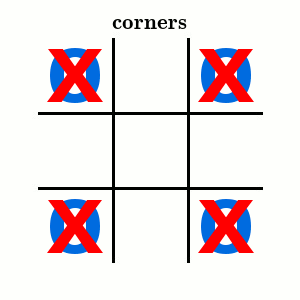The picture above should be pretty much self explanatory for the terms: Edges, Middle, Corners.

.... 1.4 Define (adjacent corner/furthest corner/adjacent edge/farther edge/ etc)

I can't really expressed in words (so I need someone else to do it, possibly Brock because he's the best)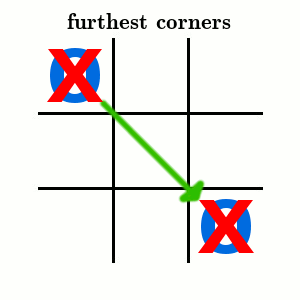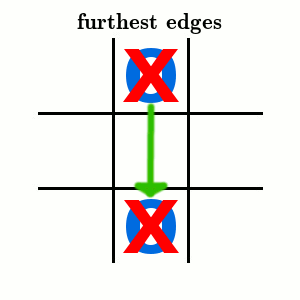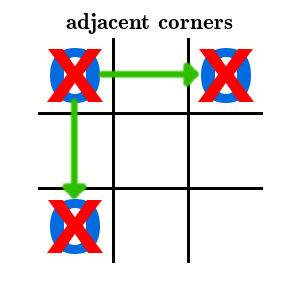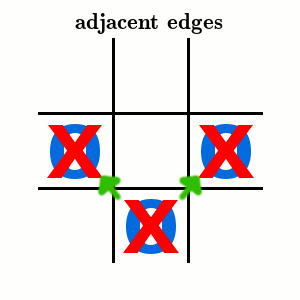# 2 Identifying forks

Define what fork is>>> you attack your oppoent in two positions and he/she can only block one and thus you can win on the next move). In other words, with a fork, you win the game. The picture in the introduction shows that Player 1 makes a fork in his third move, this is because he identified that Player 2 made a mistake on their very first move. This part explains how to see 2 or more steps ahead instead of just (one step ahead) in (section 1.2) and thus you can either avoid or create forks.

We will not show all possible forks if player 1 starts at an edge because there is too many cases, however, will be give a couple of examples to elaborate what we're saying.

Case 1:

(needs rephrasing) As shown for the introductory example, if player 1 places his X in the corner, and player 2 places his O in the opposite corner, player 1 forces player 2 to place his O in bottom middle edge and thus setting up a fork in his third move. In other words, player 1 should identify that player 2 places his O in the wrong place (opposite edge to X) from the start. Synopsis: If player 2 places his X in a corner, and player 2 places his O in the opposite corner, player 1 wins. So as player 2, you should not place your starting O that is directly opposite corner to player 1's corner O.

Case 2:(needs rephrasing) Refer to the Gif above. The setup is as follow. Player 1 places X in the middle, player 2 follows up with placing O on the edge, then player 1 reacts by placing his X to the adjacent corner to O (either left or right, does not matter), thus forcing player 2 to defend in the bottom corner, lastly player 1 can make a fork by placing X in the other bottom corner thus attacking at two fronts.

So as player 1, if you start in the middle, you should identify that you can mark a fork once player 2 places his first move on the edge; and as player 2, to avoid this fork, that is, if player 1 starts in the middle, you should always place your first move in the corner.

Case 3: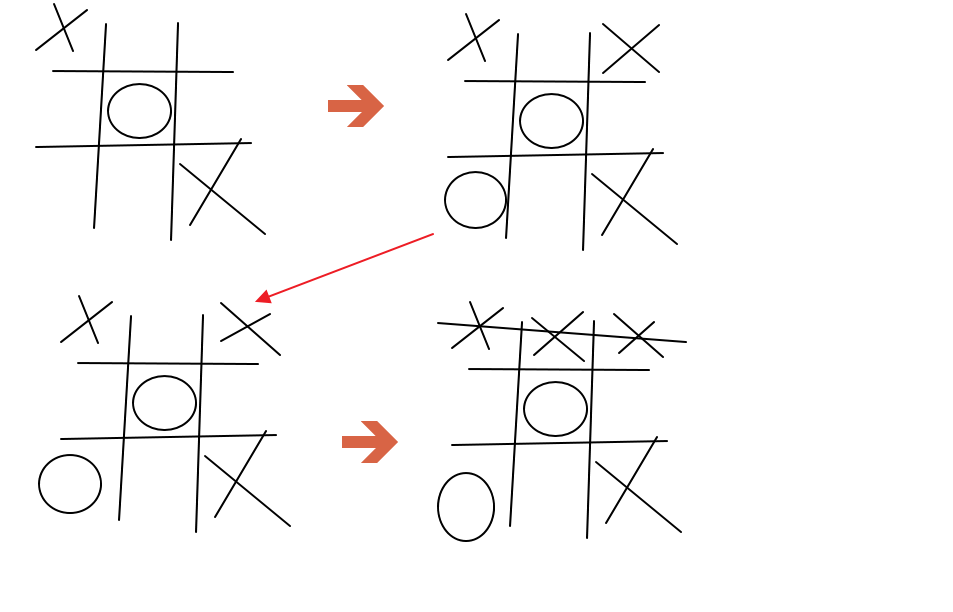(Needs rephrasing). Refer to the Gif above, the setup is as follow. Player 1 places his first move in a corner and player 2 places his first move in the middle follow by player 1 places his second move in the opposite corner. In this case, it is player 2's mistake of placing his second move in another corner as he not only forces player to place their X in the remaining corner but also inadvertly causes player 1 to create forks.

So as player 2, for the setup as such this, you should place your second move in an edge instead of a corner.

Case 4:(Needs rephrasing). Refer to the Gif above, the setup is as follow. Player 1 places his first move in the corner, and player 2 follows up by placing their first move in the adjacent edge next to player 1. Then player 1 place his next move on the middle and thus forces player 2 to block at the bottom corner, then player 1 forks by placing his third X in the other bottom corner.

So as player 1, if you start at a corner, and player 2 follows up by placing his O in the adjacent edge to you, you should identify that you can make a fork, as for player 2, you should not place your first move on the adjacent edge if player 1 makes his first in the corner.

Case 5:(Needs rephrasing). Refer to the Gif above, the setup is as follow. Player 1 places his first move in the corner, and player 2 follows up by placing their first move in the opposite edge next to player 1. Then player 1 place his next move on the middle and thus forces player 2 to block. This both forces player to block and creates a fork like in Case 4.

So as player 1, if you start at a corner, and player 2 follows up by placing his O in the opposite edge to you, you should identify that you can make a fork, as for player 2, you should not place your first move on the opposite edge if player 1 makes his first in the corner.

Case 6:(Needs rephrasing). Refer to the Gif above, the setup is as follow. Player 1 places his first move in the corner, and player 2 follows up by placing their first move in the adjacent corner next to player 1. Then player 1 place his next move in the opposite corner from his first move. This forces player 2 to block at the middle. and this in turn makes player both blocks at the remaining corner and creates a fork.

So as player 1, if you start at a corner, and player 2 follows up by placing his O in the adjacent corner to you, you should identify that you can make a fork, as for player 2, you should not place your first move on the adjacent corner if player 1 makes his first in the corner.

Case 7:(Needs rephrasing). Refer to the Gif above, the setup is as follow. Player 1 places his first move in an edge and player 2 places his first move in the adjacent edge. then player 1 follows up by placing his second move to the adjacent edge of player 2's move.

So as player 2, for the setup as such this, do not place your second move to the opposite corner to your first move.

Case 8:(needs rephrasing) Refer to the gif above, the setup is as follows. This is the extension to the introductory example. If Player 1 places in the middle, then player 2 places in the corner, then player 1 follows up by placing his move in the opposite corner to player 2. Player 2 then makes places his O in the farther edge from his first move, then player 1 can make a fork by placing his move in the opposite corner to player 2's last move.

So as player 1, you should identify that if player 2 makes his 2nd move as such, then you can make a fork. And as player 2, you should not place your 2nd move there.

Case 9: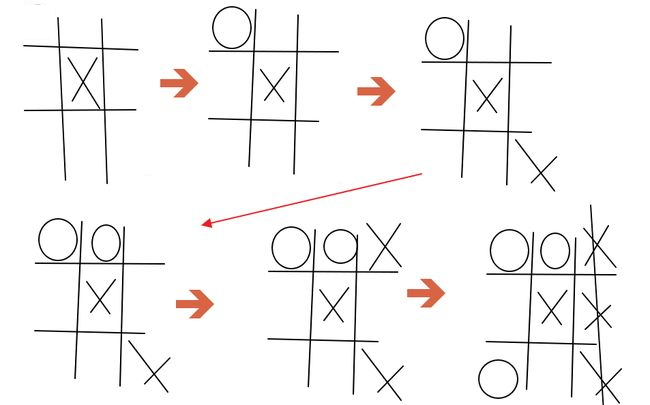(needs rephrasing) Refer to the gif above, the setup is as follows. This is the extension to the introductory example. If Player 1 places in the middle, then player 2 places in the adjacent edge to his first move, then player 1 is both force to block player 2 and creates a fork.

So as player 2, do not place your 2nd move there.

Case 10:(needs rephrasing) Refer to the gif above, the setup is as follow. If Player place on an edge and player 2 follows up by placing on an adjacent edge. then Player 1 should be able to identify a fork by placing his 2nd move in the middle thus forcing player 2 to block and lastly, player 1 places his move in a corner that is both adjacent to both players' first move.

So as player 1, if you place your first move in the edge, identify a fork can occur if player 2 place their first move on the adjacent edge, and as player 2, do not place your first move on an adjacent edge to player 1's first move as on an edge.

Case 11: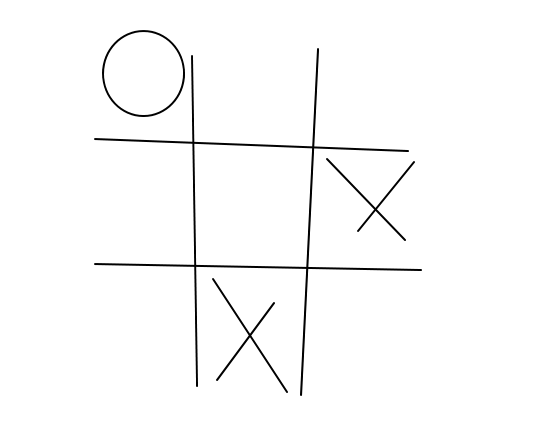(needs rephrasing) If player 2 places his 2nd move in the middle or the opposite corner from his first move, then player 1 is forced to block and creates a fork. So as player 2, for this setup, your next move is to place your O in the respective row or column to force a move from Player 1.

Case 12: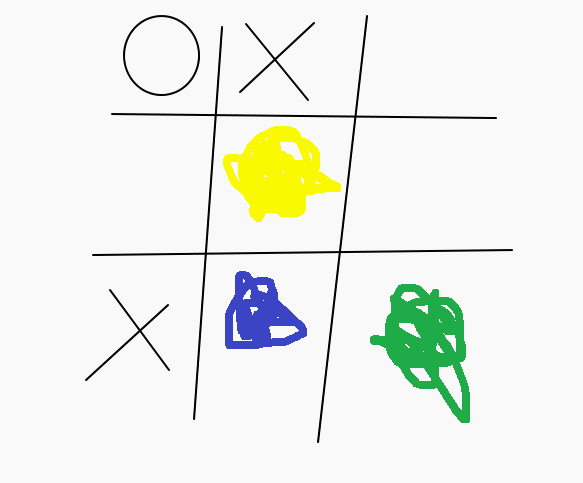(needs rephrasing) This is an extension to case 6. If player 1 places at an edge and player 2 then places at an adjacent corner to player then player 1 places his 2nd move on a corner that is adjacent to the row to player 2's first move but not adjacent to player 1's first move, then if player 2 don't place their 2nd move in any of the colored region, player 1 can forks at (blue).

Case 13:(to be filled in) so as player 1, don't place 2nd move there. and as player 2, attack diagonally.

# 3 Algorithm to play:

3.1 Based on the few case mention, we can summarize it as:

Suppose P1 choose corner, then P2 MUST choose middle, else he lose (see case 1,4,5,6). > then for a possibility of 2nd step ahead, player 1 must place in opposite corner, then P2 must avoid by placing his 2nd move on an edge (see case 3) >> forces draw.

Suppose P1 choose middle, then P2 MUST choose corner, else he lose (see case 2) > then for a possibility for a 2nd step ahead, P1 chooses a corner opposite to P2 first move > then to prevent forking >>> then P2 must place his 2nd move in another corner (see case 8 and 9) >> forces draw.

Suppose P1 choose edge, then P2 can either:
............. choose middle > P1 choose adjacent edge to 1st move > P2 choose any of the 3 corners that are adjacent to P1's X >> forces draw
............. choose middle> P1 choose furthest corner to 1st move > P2 removes fork at one of three forks (2 adjacent corners of P1's first move, or adjacent edge to P1's first and 2nd move) ............. choose adjacent corner, then P1 should not choose furthest edge from P1's first move else lose (see case 10).

3.2: To simplify the algorithm, we can adopt the following strategy

If First player takes middle, second player then takes corner


Or

If First player takes corner, second player then takes middle.


Or

If First player takes edge, second player then takes middle, then first player can place anywhere but the furthest edge, then second player then removes forking position (like in case 12).


In other words, whoever does not follow / "break off" from any of the first two algorithm loses, and players should beware of potential forks for "starting in P1 starting in edge".

- 5 years, 10 months ago

Log in to reply

1) Converting to (ax^2+bx+c==0 mod p ) <==> x^2 == a mod p, show that all quadratic congrunces can be simplified into this form.

2) Define what QR and QNR are and it's up to (p-1). so x^2 =a mod p has either 0 or 2 solutions for p \not \div a.

3) Listing out the quad residues of 13 (like in practice) show that (x^2=5mod13) has no solution

4) table of quadratic residues (1 to 25) maybe?

5) statement of Euler's Criterion, Solve x^2=5mod13 by Euler's criterion

6) Multiplicative Property statement: x^2=a mod p, x^2=b mod p, x^2=ab mod p (1 or 3 is true)

7) Write an example to prove the multiplicative property. Apply Fermat's two square identities maybe?

8) Extension from above::: listing out the quad residues of 13: (1/13) = (3/13) = (4/13) ... = (12/13) = 1, (2/13) = (5/13) = (6/13) = ... =(11/13) = -1

9) briefly define Legendre Symbol, and solve x^2=5mod13 by Legendre Symbol, some properties: a==b mod p, (a/p) =(b/p)

10) briefly define LOQR, List of some property of LOQR , solve x^2=5mod13 by LOQR

11) Mention without proof: there is precisely (p-1)/2 positive QR and QNR of odd p.

12) State theorem of Gauss Lemma and solve (x^2 = 5 mod 13) by Gauss Lemma

13) Briefly touch about: (-1/p) =\begin{cases} (1 if p==1 mod 4), (-1/p) = (-1 if p==3 mod 4), alternatively, (-1/p) = (-1)^((p-1)/2)

14) Briefly touch about: (2/p) = \begin{cases}(1 if p== \pm 1 mod 8), (2/p) = (-1 if p==\pm 3 mod 8), alternatively, (2/p) = (-1)^((p^2-1)/8)

15) Briefly touch about: (3/p) = \begin{cases} (1 if p== \pm 1 mod 12) , (-1 if p==\pm 5 mod 12)

16) Summarize by showing that we can convert to familiar notations like (-1/q), (1/q), (2/q), (3/q). And show a relevant example. E.g: (29/53) = (53/29) = ... =(2/29)(3/29) =. ... 1

17) mention that if m = any perfect squares less than p , then (m/p) = 1 (same thing as: mentioning that (4/p) = 1 which seems obvious)

18) If A is a QR, then so is k^2 A (yes, it’s duh, but it’s a basic property that’s good to know) E.g. 8/p =?

19) Find a suitable example which can utilize all (or most of the techniques): e.g: $x^2 \equiv 60 \pmod{677}$. (answer = there exist solution)

20) Briefly touch about: Composite modulo: $x^2 == a \pmod p^n$ has solution when (a/p) = 1

21) No proof: Some properties of composite modulo: $x^2 \equiv a \pmod 2$ always has solution, $x^2 \equiv a \pmod 4$ has solution of $a\equiv1\pmod4$, $x^2\equiv a\pmod{2^n}$ for n>=3 and a ==1 mod8.

22) Theorem for composite modulo: Let n = 2^k0 x product(p1^k1 x .. x pr^kr ) for n>1 with gcd(a,n) = 1, then x^2==a mod n is solvable IFF (a/pi) = 1 for i=1,2,3,...,r, and a==1 mod4 if 4 divides n but 8 don't divide n, a == 1 mod 8 if 8 | n.

23) Relevant example for (23) Solve the congrunce x^2==31 mod 114; determine number of solutions of congruences x^2==3 mod (11*23)^2, x^2 ==9 mod(8 * 3 * 25)

24) An example or two: Find the least quadratic nonresidue of modulo (p). Some p.

25) Use the example (37 | (x^2-31x-34) ) <<< completing the square. Main purpose = identify what residue we should be looking out for. That is $x^2 = a \pmod p$ ==> a = ?

- 5 years, 10 months ago

Log in to reply

Possible flow chart::::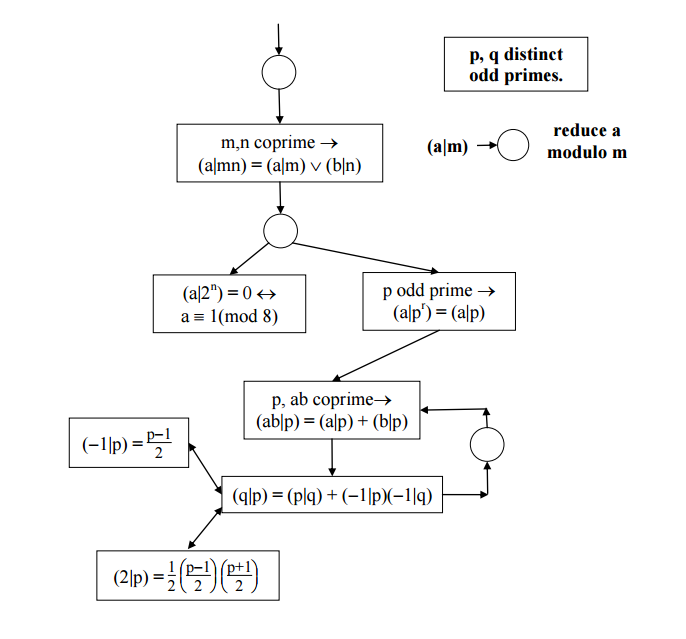link.

- 5 years, 10 months ago

Log in to reply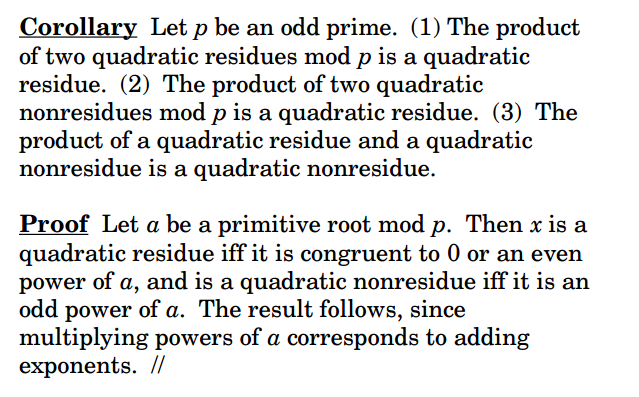- 5 years, 10 months ago

Log in to reply

×

Problem Loading...

Note Loading...

Set Loading...